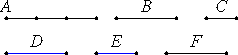# Proposition 6

If two magnitudes have to one another the ratio which a number has to a number, then the magnitudes are commensurable.

Let the two magnitudes A and B have to one another the ratio which the number D has to the number E.

I say that the magnitudes A and B are commensurable.

Divide A into as many equal parts as there are units in D, and let C equal one of them, and let F be made up of as many magnitudes equal to C as there are units in E.VII.Def.20

Since then there are in A as many magnitudes equal to C as there are units in D, whatever part the unit is of D, the same part is C of A also. Therefore C is to A as the unit is to D.

V.7,Cor.

But the unit measures the number D, therefore C also measures A. And since C is to A as the unit is to D, therefore, inversely, A is to C as the number D is to the unit.

VII.Def.20

Again, since there are in F as many magnitudes equal to C as there are units in E, therefore C is to F as the unit is to E.

V.22

But it was also proved that A is to C as D is to the unit, therefore, ex aequali, A is to F as D is to E.

V.11

But D is to E as A is to B, therefore A is to B as it is to F also.

V.9

Therefore A has the same ratio to each of the magnitudes B and F. Therefore B equals F.

But C measures F, therefore it measures B also. Further it measures A also, therefore C measures A and B.

X.Def.1

Therefore A is commensurable with B.

Therefore, if two magnitudes have to one another the ratio which a number has to a number, then the magnitudes are commensurable.

Q.E.D.

# Corollary

VI.9

From this it is clear that, if there are two numbers as D and E, and a straight line as A, then it is possible to make a straight line F such that the given straight line is to it as the number D is to the number E.

VI.19,Cor.

And if a mean proportional is also taken between A and F, as B, then A is to F as the square on A is to the square on B, that is, the first is to the third as the figure on the first is to that which is similar and similarly described on the second.

But A is to F as the number D is to the number E, therefore the number D is to the number E as the figure on the straight line A is to the figure on the straight line B.

Q.E.D.

## Guide

If A : B = m : n, then, with C equal to A/m, it follows that A = mC and B = nC.

The proof assumes that magnitudes are divisible. Not all magnitudes, however, are constructively divisible. For instance, a 60° angle cannot be trisected by a Euclidean construction. An alternate proof which does not depend on divisibility of magnitudes can be based on antenaresis.

Although the proposition refers to magnitudes of any kind, the corollary only applies to lines in the first statement and plane figures in the second.

#### Use of this proposition

The proposition is used in very frequently in Book X starting with the next proposition, its contrapositive. It is also used in proposition XIII.6. The corollary is also used frequently in Book X starting with X.10.